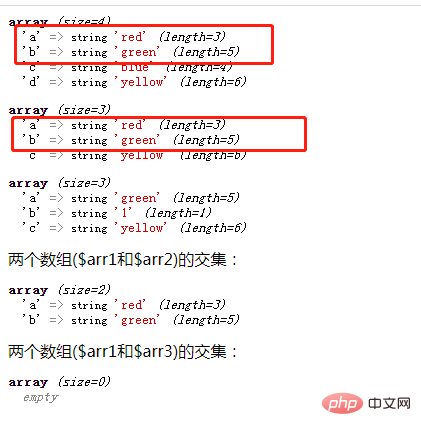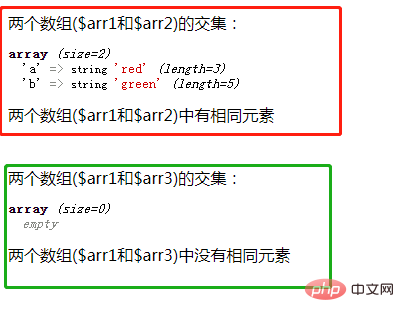# php怎么对比两个数组是否有相同元素php对比两个数组是否有相同元素的方法

array_intersect_assoc() 函数用于比较两个数组的键名和键值，并返回两个数组的交集（包含相同元素的数组）。

```<?php
\$arr1=array("a"=>"red","b"=>"green","c"=>"blue","d"=>"yellow");
\$arr2=array("a"=>"red","b"=>"green","c"=>"yellow");
\$arr3=array("a"=>"green","b"=>"1","c"=>"yellow");
var_dump(\$arr1);
var_dump(\$arr2);
var_dump(\$arr3);
\$result=array_intersect_assoc(\$arr1,\$arr2);
echo '两个数组(\$arr1和\$arr2)的交集：';
var_dump(\$result);
\$result=array_intersect_assoc(\$arr1,\$arr3);
echo '两个数组(\$arr1和\$arr3)的交集：';
var_dump(\$result);
?>```• 如果交集数组为空数组，则两个数组里没有相同元素

• 如果交集数组不是空数组，则两个数组里有相同元素

```if(\$result==[]){
echo '两个数组中没有相同元素<br>';
}else{
echo '两个数组中有相同元素<br>';
}```1/1